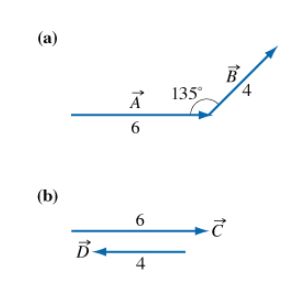# Problem: A) What is the magnitude of the cross product AxB?B) What is the direction of the cross product AxB? - into the page? - out of the page? - the magnitude is zero? C) What is the magnitude of the cross product CxD ?D) What is the direction of the cross product CxD? - into the page? - out of the page? - the magnitude is zero?

###### FREE Expert Solution

The magnitude of a cross product is given by:

$\overline{){\mathbf{A}}{\mathbf{×}}{\mathbf{B}}{\mathbf{=}}{\mathbf{A}}{\mathbf{B}}{\mathbf{s}}{\mathbf{i}}{\mathbf{n}}{\mathbf{\theta }}}$, where θ is the angle between vector A and B.

84% (227 ratings)###### Problem DetailsA) What is the magnitude of the cross product AxB?

B) What is the direction of the cross product AxB? - into the page? - out of the page? - the magnitude is zero?

C) What is the magnitude of the cross product CxD ?

D) What is the direction of the cross product CxD? - into the page? - out of the page? - the magnitude is zero?